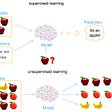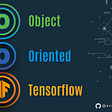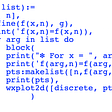# Inner Product

`struct InnerProdFIR {     int zPtr = 0; // state pointer     float z[FILTER_ORDER]; // filter state     float h[FILTER_ORDER]; // filter kernelvoid process (float* buffer, int numSamples)     {         float y = 0.0f;         for (int n = 0; n < numSamples; ++n)         {             z[zPtr] = buffer[n]; // insert input into state// compute two inner products between kernel and wrapped state buffer             y = std::inner_product (z + zPtr, z + FILTER_ORDER, h, 0.0f);             y = std::inner_product (z, z + zPtr, h + (FILTER_ORDER - zPtr), y);zPtr = (zPtr == 0 ? FILTER_ORDER - 1 : zPtr - 1); // iterate state pointer in reversebuffer[n] = y;         }     } };`

# Double-Buffered Inner Product

`struct InnerProdNoWrapFIR {     int zPtr = 0; // state pointer     float z[2 * FILTER_ORDER]; // filter state     float h[FILTER_ORDER]; // filter kernelvoid process (float* buffer, int numSamples)     {         float y = 0.0f;         for (int n = 0; n < numSamples; ++n)         {             // insert input into double-buffered state             z[zPtr] = buffer[n];             z[zPtr + FILTER_ORDER] = buffer[n];// compute inner product over kernel and double-buffer state             y = std::inner_product (z + zPtr, z + zPtr + FILTER_ORDER, h, 0.0f);zPtr = (zPtr == 0 ? FILTER_ORDER - 1 : zPtr - 1); // iterate state pointer in reversebuffer[n] = y;         }     } };`

# SIMD Optimization

`// inner product using SIMD registers inline float simdInnerProduct (float* in, float* kernel, int numSamples, float y = 0.0f) {     constexpr size_t simdN = dsp::SIMDRegister<float>::SIMDNumElements;// compute SIMD products     int idx = 0;     for (; idx <= numSamples - simdN; idx += simdN)     {         auto simdIn = loadUnaligned (in + idx);         auto simdKernel = dsp::SIMDRegister<float>::fromRawArray (kernel + idx);         y += (simdIn * simdKernel).sum();     }// compute leftover samples     y = std::inner_product (in + idx, in + numSamples, kernel + idx, y);return y; }`

# Finally

--

--

--

## More from Jatin Chowdhury

Jatin Chowdhury is a student.

Love podcasts or audiobooks? Learn on the go with our new app.

## LINUX `ls` commands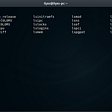## Setting Up Raspberry Pi 4 3.5" Touch Screen XPT2046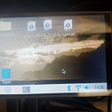## The Guide to Docker ARG, ENV and .env## Let’s Automate :: Let’s Encrypt TLS Certs for OpenShift 4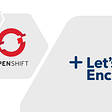## What makes Open Source CRM better than Free CRM## Software Development is the Most Disappointing Job Ever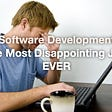## Kulkul Event this December## Xamarin Forms: how to troubleshoot Android crashes where all you see is Java/Android native code…## Jatin Chowdhury

Jatin Chowdhury is a student.

## The Black Box Analogy: Theoretical Explanation